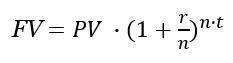# Future Value Calculator

Use this FV calculator to easily calculate the future value (FV) of an investment of any kind. A versatile tool allowing for period additions or withdrawals (cash inflows and outflows), a.k.a. future value with payments. Computes the future value of annuity by default, but other options are available.

\$
%
Share calculator:

Embed this tool:
get code

## Using the future value calculator

This financial calculator can help you calculate the future value of an investment or deposit given an initial investment amount, the nominal annual interest rate and the compounding period. Optionally, you can specify periodic contributions or withdrawals and how often these are expected to occur.

The output of the FV calculator consists of:

• the final value of the investment
• the present value (PV) of the investment
• total interest accrued, effective interest rate, capital growth, and more

## What is "Future value"?

Future value represents the value of a given investment at a specified point in the future, assuming that you are able to grow it at a given rate per period and accounting for compounding, contributions or withdrawals, and when they happen. It is useful when you want to estimate the pay off from an investment with a given present value by taking the time value of money into account. The investment could be a deposit in a savings account, a business project, stock market portfolio, investment fund, etc.

Using the future value calculator can help you plan and allocate resources more intelligently. Knowing the future value can help you decide between investing one way or another, or spending the money now. Like any other mathematical model, future value calculation has assumptions whose violation leads to inaccurate results. Most importantly, it assumes a steady rate of return. The result also depends on the accuracy of the predicted interest rate - even small discrepancies here can result in relatively large differences in actual results due to the compounding effect.

It should also be noted that the future value calculated is nominal: it doesn't take into account inflation or other factors that might affect the actual value of money in the future.

## Future value formula

The basic future value can be calculated using the formula:where FV is the future value of the asset or investment, PV is the present or initial value (not to be confused with PV which is calculated backwards from the FV), r is the Annual interest rate (not compounded, not APY) in decimal, t is the time in years, and n is the number of compounding periods per unit t.

This simple equation is what drives our software as well. When you enter an annual interest rate it calculates the future value of annuity, but it can be used for monthly, daily, quarterly, etc. cash flows.

## Future Value calculation example

Let us assume a \$100,000 investment with a known annual interest rate of 14% from which one wants to withdraw \$5,000 at the end of each annual period. What is the future value of this investment if we expect 1, 2, 3, 5, or 10 years from now? The answers are shown in the table below.

Future value of annuity example table
Initial ValueRate of ReturnNumber of YearsYearly PaymentPresent Value
\$100,000 14% 1 -\$5,000 \$109,000
\$100,000 14% 2 -\$5,000 \$119,260
\$100,000 14% 3 -\$5,000 \$130,956
\$100,000 14% 5 -\$5,000 \$159,491
\$100,000 14% 10 -\$5,000 \$274,036

This is a great example of how the time value of money operates. The future value of the annuity increases the more time we are willing to wait to receive it, even if the rate of return and the initial investment are exactly the same. Note the large effect of the relative small annual withdrawals (just 5% of the initial investment) - without them the FV with 10-year annuity would be \$370,722, or nearly \$100,000 on top of the value without the postponed consumption. This is why one should avoid widthrawing from a savings account and why reinvesting the interest pays off so much.

## Financial caution

This is an online tool which is a good starting point in estimating the future value of an investment and the capital growth you can expect from a bank deposit or a similar investment, but is by no means the end of such a process. You should always consult a qualified professional when making important financial decisions and long-term agreements, such as long-term bank deposits. Use the information provided by the software critically and at your own risk.

#### Cite this calculator & page

If you'd like to cite this online calculator resource and information as provided on the page, you can use the following citation:
Georgiev G.Z., "Future Value Calculator", [online] Available at: https://www.gigacalculator.com/calculators/future-value-calculator.php URL [Accessed Date: 01 Apr, 2023].